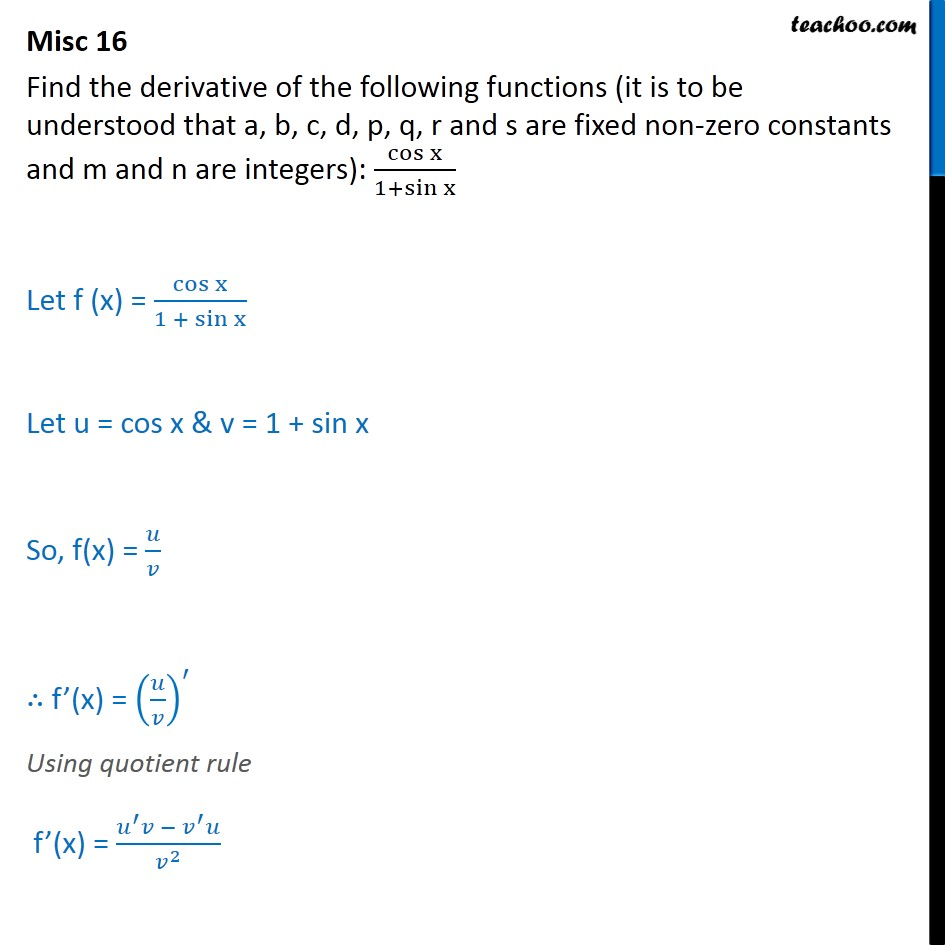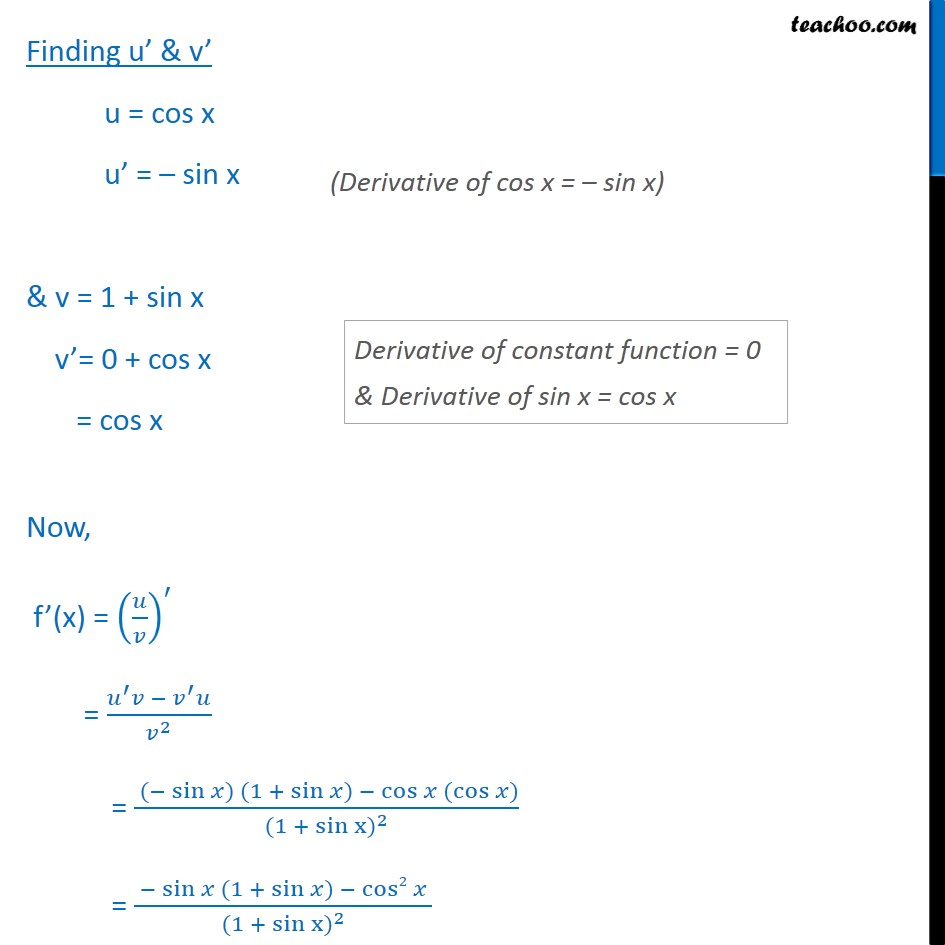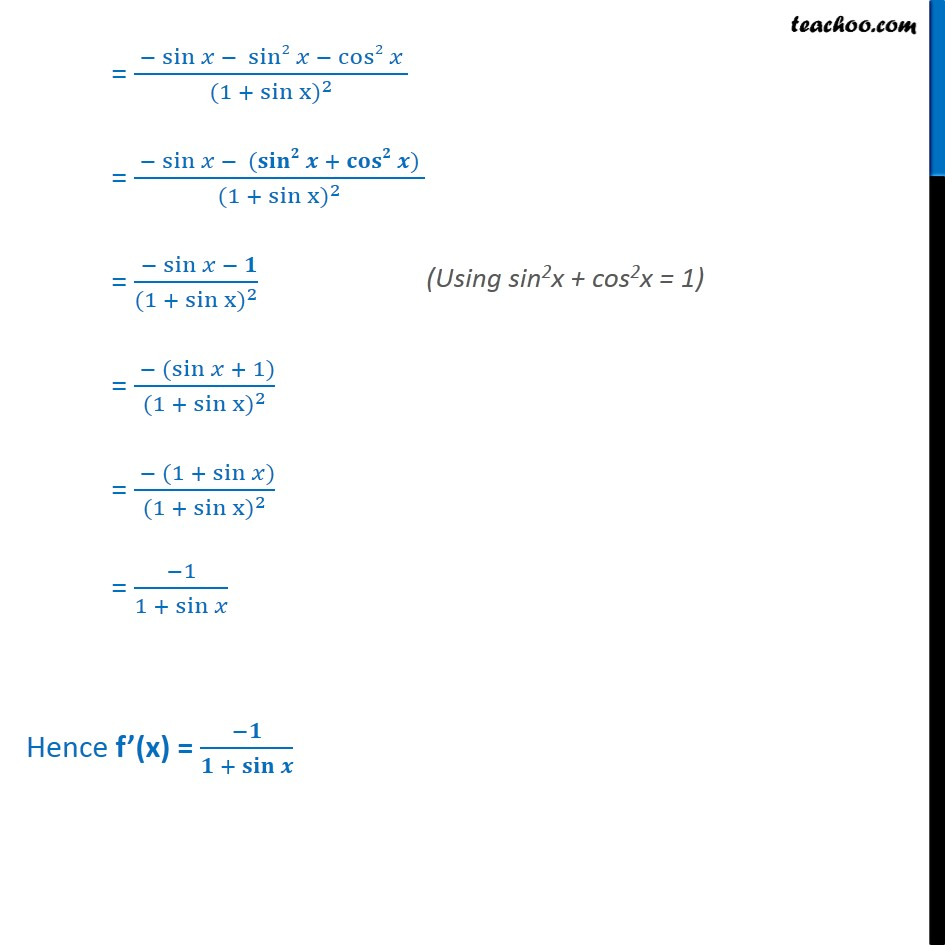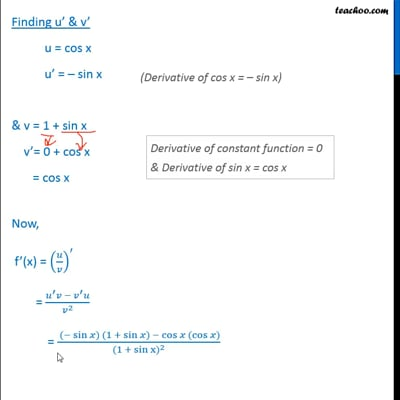Derivatives by formula - sin & cos

Chapter 13 Class 11 Limits and Derivatives
Concept wiseThis video is only available for Teachoo black users

Introducing your new favourite teacher - Teachoo Black, at only ₹83 per month

### Transcript

Misc 16 Find the derivative of the following functions (it is to be understood that a, b, c, d, p, q, r and s are fixed non-zero constants and m and n are integers): cos x 1+ sin x Let f (x) = cos x 1 + sin x Let u = cos x & v = 1 + sin x So, f(x) = f (x) = Using quotient rule f (x) = 2 Finding u & v u = cos x u = sin x & v = 1 + sin x v = 0 + cos x = cos x Now, f (x) = = 2 = ( sin ) (1 + sin ) cos ( cos ) (1 + sin x ) 2 = sin (1 + sin ) cos2 (1 + sin x ) 2 = sin sin2 cos2 (1 + sin x ) 2 = sin ( + ) (1 + sin x ) 2 = sin (1 + sin x ) 2 = (sin + 1) (1 + sin x ) 2 = (1 + sin ) (1 + sin x ) 2 = 1 1 + sin Hence f (x) = +